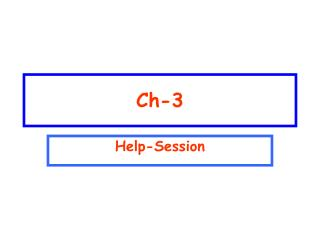DownloadDownload PresentationCh-3

# Ch-3

Download Presentation## Ch-3

- - - - - - - - - - - - - - - - - - - - - - - - - - - E N D - - - - - - - - - - - - - - - - - - - - - - - - - - -
##### Presentation Transcript

1. Ch-3 Help-Session

2. T082 Q7. Vector A has a magnitude of 40.0 cm and is directed 60.0 degrees above the negative x-axis. Vector B has magnitude of 20.0 cm and is directed along the positive x-axis. Find the resultant vector (i and j are unit vectors along positive x and y axes, respectively). (Ans: 34.6 j cm) Q8. Consider two vectors A = (3 i + 4 j) cm and B = (- 4 i + 3 j) cm. Find the angle between these two vectors. (Ans: 90 degrees) CH-3-082 A.B=ABcos =AxBx+AyBy  = cos-1[(AxBx+AyBy)/AB] A= B= 5  = cos-1[(-12+12)/ 25]= cos-1(0) = 90 degrees C=A+B; A= A cos 120 i + A sin 120 j ; A= - 20.0 i + 34.6 j B= 20 i; C=A+B = (-20+20) i + 34.6 j = 34.6 j

3. Q9. If vector A is added to vector B, the result is (6 i + 1j) m. If A is subtracted from B, the result is (- 4 i + 7 j) m. Find the magnitude of B. (Ans. 4 m.) T081 Q7. A boat travels a distance of 27.0 km 20.0o North of East. It then travels 53.0 km in a direction 30.0o East of North. What is the total distance traveled by the boat in the East direction? ( Ans: 51.9 km) CH-3-082/081 B+A= 6 i + 1j; B-A= - 4 i + 7 j; Adding both equations together 2B= 2 i + 8j B=i+4j B =(12+42)= 4 A= 27 km, A= 20 B=53 km; B=60 Cx=Ax+Bx= Acos 20 + B cos60 Cx=25.37 + 26.5 = 51.9

4. Q8. Consider two vectors A and B with magnitudes 5 cm and 8 cm, respectively. Vector A is along the positive x-axis and vector B is along the positive y-axis. Find A· (A+B). (Ans: 25 cm2) Q9. Consider the following three vectors A= 5i+ 2j-5k, B = -2i+4j+3k, and C=i-2j-3k . Find the magnitude of the vector D= A – B + 2C. (Ans: 17.7) CH-3-081 D= A-2B+3C =5i+2j-5k+2i-4j-3k+2i-4j-6k =9i-6j-14k |D|=  (92+62+142 )= 313= 17.7 A=5i; B=8j ; A+B= 5i+8j A. (A+B) = 5x5= 25

5. T072 Q5.Vectors a, b, and c are related through equations and A+B=C; A-B=5C. If C= 3i+4j, what is the magnitude of vector a ? (Ans: 15) Q6. Three vectors F,v and B are related through F = 5(vxB) . If vector v=3i -5j and B=-2k, then vector F is: (Ans: 50 i+30 j ) CH-3-072 A+B=C; A-B=5C; Adding both equations together 2A=6C; A=3C A=3C=9i+12j A  =(92+122)= 15 F = 5(vxB)=5[(3i -5j)x(-2k)] = 5[ -6i xk+10jxk)]=5[6j+10i] = 30 j+50 i

6. Q7. A vector A of magnitude 20 is added to a vector B of magnitude 25. The magnitude of the vector A+B can be: (Ans: 12) CH-3-072 Q8. Vectors F and G are defined as F=3i+4j , and G=-i+j . Find the component (projection) of vector G along the direction of vector F .( Ans: 0.20) F.G=FGcos  =FxGx+FyGy Gcos = (FxGx+FyGy)/F = (-3+4)/(32+42)=1/5 =0.2 A+Bmax =25+20=45 A+Bmin =25-20 =5 Value of A+B=5-45

7. CH-3-071 T071 :: In Fig. 3, the unknown vector C is given by: (Ans:B-A ) Q8. Two vectors are given by: P=-1.5i+2j,and Q=1.0j . The angle that the vector makes with the positive x-axis is: (A: 135°) A-C = B ; -C=B-A; C=A-B =cos-1[(PxQx+PyQy)/P   Q ] =cos-1[(2x1)/(1.52+22) x1)]

8. CH-3-071 Q5. Q9.: A man walks 5.0 km due North, then 13 km 22.6° South of East, and then 12 km due West. The man is finally at: (Ans: where he started) Add components A=5j; B=13 km 22.6 degrees south of east C= -12i Resultant must be Zero